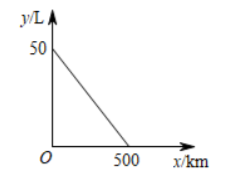$\text{A.}$ $150 km$ $\text{B.}$ $165 km$ $\text{C.}$ $125 km$ $\text{D.}$ $350 km$
【答案】 A

【解析】 【详解】解: 设函数解析式为 $y=k x+b$,

$$\left\{\begin{array}{c} b=50 \\ 500 k+b=0 \end{array}\right.$$

$\therefore$ 函数解析式为 $y=-\frac{1}{10} x+50$